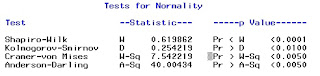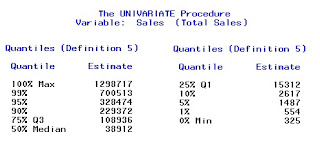## Pages

### Descriptive Statistics With Proc UnivariateBefore going to a battle, a warrior better know what he is fighting against and so a data analyst ! It is advised to know and feel the  data before carrying out analysis on it. It is the best practice to examine the data initially by using the Proc Univariate in SAS.
This is one of the procedures in SAS, that people often find quite difficult to understand. I also took quite a while to learn about it, as I first tried avoid learn it.

But  no more worries ... let's learn it and try to make it as simple as possible!

### When to use Proc Univariate?

Following are the most common points that trigger the need of Proc Univariate:

1. When you need to know basic statistical measures such as  Mean, median, range, Standard Deviation, skewness, kurtosis of a a variable in data.

2. For normality testing a variable

3. Getting percentile distribution

4.  Plotting a histogram

5. Outlier checking

### Let's see how it works!

For the sake of demo, we are using an in built data of SAS.Proc Univariate data = SASHelp.Shoes   normal;
Var sales;
Histogram sales/normal;
Run;

Let's know the syntax better >>>>>>>>>>

Run and it check the result.

### Let's understand the result!First table that we get is the moments table :

Here you get the N (no. of observations), Mean,
Standdard Deviation, kurtosis etc.

We also get coefficient of variation which is (Standard Deviation / Mean).

Skewness : It is degree and direction of a data being asymmetric .

A positive (right) skewed data means that there are few extreme large observations which make its mean to skew positively. Here Mean is greater than median and median is greater than mode.

A negative (left) skewed data means that there are few extreme small observations which make its mean to skew negatovely. Here Mean is less than median and median is less  than mode.The second table gives additional information of  Median, Mode and Inter-quartile range ( Which is 75% percetile - 25% percentile).

The table itself gives and idea of distribution of variable. A normally distributed data has Mean, Median and Mode quite close to each other.

The third table is result of hypothesis testing where mean of variable is being tested against 0.p-value quite less that 0.05 means that we can reject the null hypothesis of mean being equal to 0 and hence mean is quite different from 0.

There are three independent statistical test for testing the same hypothesis.

The fourth table comes in the output only when you use option "normal" in the syntax.
Here you get a proper statistical evidence of data being normal or not normal. There are 4 tests of normality.For a relatively small sample (upto 2000 observations), we check the first test (Shapiro Wilk) and see if the p value. If p value is less that 0.05 then data is not normal . Shapiro-Wilk test state the null hypothesis of normality, with p value less that 0.05, we reject the null hypothesis. Data is normal for more than 0.05 p value.

For large samples (more than 2000 observations), we generally use Kolmogorov-Smirnov Test.

For Kolmogorov-Smirnov Test too, the null hypothesis states that data is normal and hence if p value should be more than 0.05 for data being normal. Rest two test are also similar.

The fifth table (often in two parts) gives the percentile distribution in a fixed format:We can also take output at customized percentile points, which we are showing later in the article itself.

But this table also gives a fair idea about the data, how it is distributed, Also looking at the extreme deciles, we can get an idea of having outliers.The last (sixth) table contains the top and bottom 5 values of the variable.Additionally we get a Histogram of the variable which explains the distribution best visually.

As they say .... "a picture is worth a thousand words"

The histograms says it all, whether it is normally distributed or not, whether there are outlier or not.

Here data is right (positive) skewed and not following a normal distribution.

### Generate 10th, 20th, 30th ..... 9th, 100th percentile

Proc Univariate data = SASHelp.Shoes noprint;
var sales ;
output out = percentile
Pctlpts = 10 20 30 40 50 60 70 80 90 100   Pctlpre = P_;

Run;

Run the code and check the data ... you get your required result.

You can also write in in following fashion :

Let's see one more variation in the syntax :

Proc Univariate data = SASHelp.Shoes   plots;
Var sales;
Run;

The code, in addition to above explained things, gives few additional things :

1.  Stem and Leaf Plot along with a Box Plot
2. Normal Probability Plot

It would take another article to explain the things, which we will do for sure real soon!

For now you can use the following link to better understand the same. Also you can get a lot of theory ...so enjoy learning.

### Annotated Output of Proc Univariate

Enjoy reading our other articles and stay tuned with us.

Kindly do provide your feedback in the 'Comments' Section and share as much as possible.

1.2.3.4.5.6.